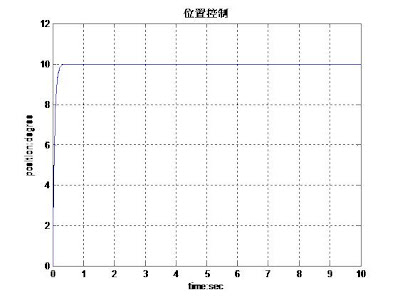## 2011年7月28日 星期四

### 粒子群演算法尋找比例控制器P最佳參數

y(s)/u(s)=(s+1)/(s^2+2s+1)...................(1)(圖一)

PID控制器對於受控對象系統模型不明確仍有極佳的控制效能，因此廣泛使用於工業控制。然而PID雖然結構簡單，但如果參數設定不佳，仍有可能使系統產生不佳的控制效果，甚至造成系統的不穩定。

[程式碼]
`clear allclc%初始化N=200;         %partical 個數Period=10;   % 迭代次數Vmax=1;kp=15*rand(1,N)+5;            %產生N個數的kp隨機值，範圍介於+5~+20P_best=kp;                    %個體最優值，初始狀態取kp相同值G_best=25;V=zeros(1,N);C1=2;C2=2;W=0.8;for i=1:Periodifor j=1:N    V(2,j)=W*V(1,j)+C1*rand(1)*(P_best(1,j)-kp(1,j))+C2*rand(1)*(G_best-kp(1,j));    if(abs(V(2,j)>Vmax))        V(2,j)=sign(V(2,j))*Vmax;    end    kp(2,j)=kp(1,j)+V(2,j);    P_best_fitness=PSO_position_control(P_best(1,j));    current_particle_fitness=PSO_position_control(kp(2,j));    if(current_particle_fitness<P_best_fitness)        P_best(1,j)=kp(2,j);    endendV(1,:)=V(2,:);kp(1,:)=kp(2,:);for j=1:N    P_best_fitness=PSO_position_control(P_best(1,j));    G_best_fitness=PSO_position_control(G_best);    if(P_best_fitness<G_best_fitness)        G_best=P_best(1,j);    endendendG_best`

[副函式PSO_position_control.m]
`function fitness=PSO_position_control(kp)%系統轉移函數  sys_open(s)=(s+1)/(s^2+2s+1)num=[1 1];den=[1 2 1];sys_open=tf(num,den);%轉移函數轉換為狀態空間方程式[a,b,c,d]=tf2ss(num,den);sys_open=ss(a,b,c,d);%初始設定Ts=0.01;       %sampling timex=zeros(2,1);  %state space variables is set to zerou=0;R=10;          %reference valuey=0;           %current state space output value, assume as velocityposition=0;    %current positioncnt=1;err=0;         %error between reference position value and current one.sum_err=0;for t=0:Ts:10 dot_x=a*x+b*u; y=c*x+d*u; position=position+y*Ts; err=R-position; sum_err=sum_err+0.5*(err)^2; u=kp*err; x=dot_x; plot_position(cnt)=position; cnt=cnt+1;endfitness=sum_err;`

[執行結果]（圖二）

## 2011年7月25日 星期一

### 求解最佳化的問題-使用粒子群演算法,part4

particle 個數N=200

Vmax=5粒子飛行速度最大值

X=10*rand(1,N)-5，隨機產生N個-5~+5之間的數

V＝zeros(1,N)，所有粒子飛行速度初值均為0

C1=C2=2

W=0.8

G_best=1000，寫大一點，否則會一下子就收斂

P_best=X ，一開始，個體的最佳值即一開始當前的位置

[程式碼]

`%一維函數F(x)=x^2，給定範圍找最小值clear allclc%初始化N=200;         %partical 個數Period=1000;   % 迭代次數Vmax=5;X=10*rand(1,N)-5;            %產生N個數的隨機值，範圍介於-5~+5P_best=X;                    %個體最優值，初始狀態取X相同值G_best=1000;V=zeros(1,N);C1=2;C2=2;W=0.8;cnt=1;for i=1:Period    for j=1:N        V(2,j)=W*V(1,j)+C1*rand(1)*(P_best(1,j)-X(1,j))+C2*rand(1)*(G_best-X(1,j));        if(abs(V(2,j)>Vmax))            V(2,j)=sign(V(2,j))*Vmax;        end        X(2,j)=X(1,j)+V(2,j);        P_best_fitness=fitness(P_best(1,j));        current_particle_fitness=fitness(X(2,j));        if(current_particle_fitness<P_best_fitness)            P_best(1,j)=X(2,j);        end    end    V(1,:)=V(2,:);    X(1,:)=X(2,:);    for j=1:N        P_best_fitness=fitness(P_best(1,j));        G_best_fitness=fitness(G_best);        if(P_best_fitness<G_best_fitness)            G_best=P_best(1,j);        end    end    G_best_dot(cnt)=G_best;    cnt=cnt+1;endplot(G_best_dot)G_best`

`副函數fitness:function fit=fitness(X)    fit=X^2;end [執行結果]從圖中可看出最佳值收斂，且經計算得到G_best=-3.9680e-021換句話說，電腦分析當-3.9680e-021可得函數F(-3.9680e-021)的最小值。當然，迭代的分析，必然存在誤差，但其結果仍令人滿意，與期望值0極為接近。`

### 求解最佳化的問題-使用粒子群演算法,part3

1.每一粒子飛行速度與方向遷就於粒子本身及群體，定義飛行速度：

Vi(k+1)=W*Vi(k)+C1*rand()*(P_best_i-Xi(k))+C2*rand()*(G_best-Xi(k))............(1)

W是權重常數，考慮前一刻飛行速度，對下一刻的飛行速度的影響程度

Vi(k+1)：第i個粒子，第k+1時刻的飛行速度

Vi(k)：第i個粒子，第k時刻的飛行速度

C1,C2：學習係數，當前位置與最佳位置，影響下一時刻飛行速度的權重

rand()：隨機函數，產生0~1之間的小數

P_best_i：第i個粒子，所走路徑中自始至今之最佳值

G_best：群體粒子之最佳值

Xi(k)：第i個粒子，當前所在位置

2.定義粒子下一時刻的位置：

Xi(k+1)=Xi(k)+Vi(k+1)...........................(2)

Xi(k+1)：第i個粒子，在第k+1時刻的位置

Xi(k)：第i個粒子，在第k時刻的位置

(2)式，速度‧時間＝Vi(k+1)△t=位置

3.第i粒子最佳位置取代原則：

4.群體最佳值的修正時機：

（待續）

### 求解最佳化的問題-使用粒子群演算法,part1dF(x)/dx=2x....................(2)

## 2011年7月15日 星期五

### 數位I/O與邏輯分析器的常用名詞及定義

http://zone.ni.com/devzone/cda/tut/p/id/6247